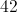# High School Math : How to find the perimeter of a hexagon

## Example Questions

### Example Question #1 : How To Find The Perimeter Of A Hexagon

What is the perimeter of a regular hexagon with a side length of 12?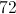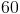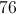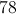Explanation:

To find the perimeter of a regular hexagon you must first know the number of sides in a hexagon which is 6.

When you know the number of sides of a regular polygon to find the perimeter you must multiply the side length by the number of sides.

In this case it is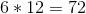The answer for the perimeter is.

### Example Question #2 : How To Find The Perimeter Of A Hexagon

How many degrees is the interior angle of any regular hexagon?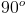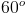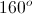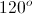Explanation: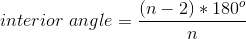To find the angle of any regular polygon, you find the number of sides,. In this example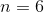.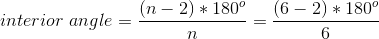You then subtractfrom the number of sides, yielding.

Takeand multiply it by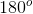to yield the total number of degrees in the regular hexagon.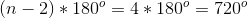Then, to find one individual angle, we divide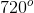by the total number of angles,.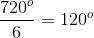The answer is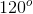.

### Example Question #3 : How To Find The Perimeter Of A Hexagon

What is the perimeter of a hexagon with a side length of?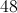Explanation:

To find the perimeter of a regular polygon, we take the length of each side,, and multiply it by the number of sides,.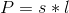In a hexagon the number of sides is, and in this example the side length is.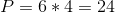The perimeter is.

### Example Question #4 : How To Find The Perimeter Of A Hexagon

How many lines of symmetry can be found in a regular hexagon?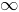Explanation:

The number of lines of symmetry through a regular polygon is equal to the number of sides.

A hexagon has lines of symmetry through each vertex, giving three lines of symmetry that each connect two opposite vertices. The other three lines pass through the midpoints of opposite sides of the hexagon.

### Example Question #5 : How To Find The Perimeter Of A Hexagon

What is the perimeter of a regular hexagon with a side length of?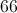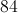Explanation:

To find the perimeter of a regular hexagon you must first know the number of sides in a hexagon which is.

When you know the number of sides of a regular polygon to find the perimeter you must multiply the side length by the number of sides.

In this case it isThe answer for the perimeter is.

### Example Question #6 : How To Find The Perimeter Of A Hexagon

This figure is a regular hexagon with one side measuring 6 cm.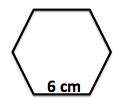What is the perimeter of the regular hexagon in centimeters?